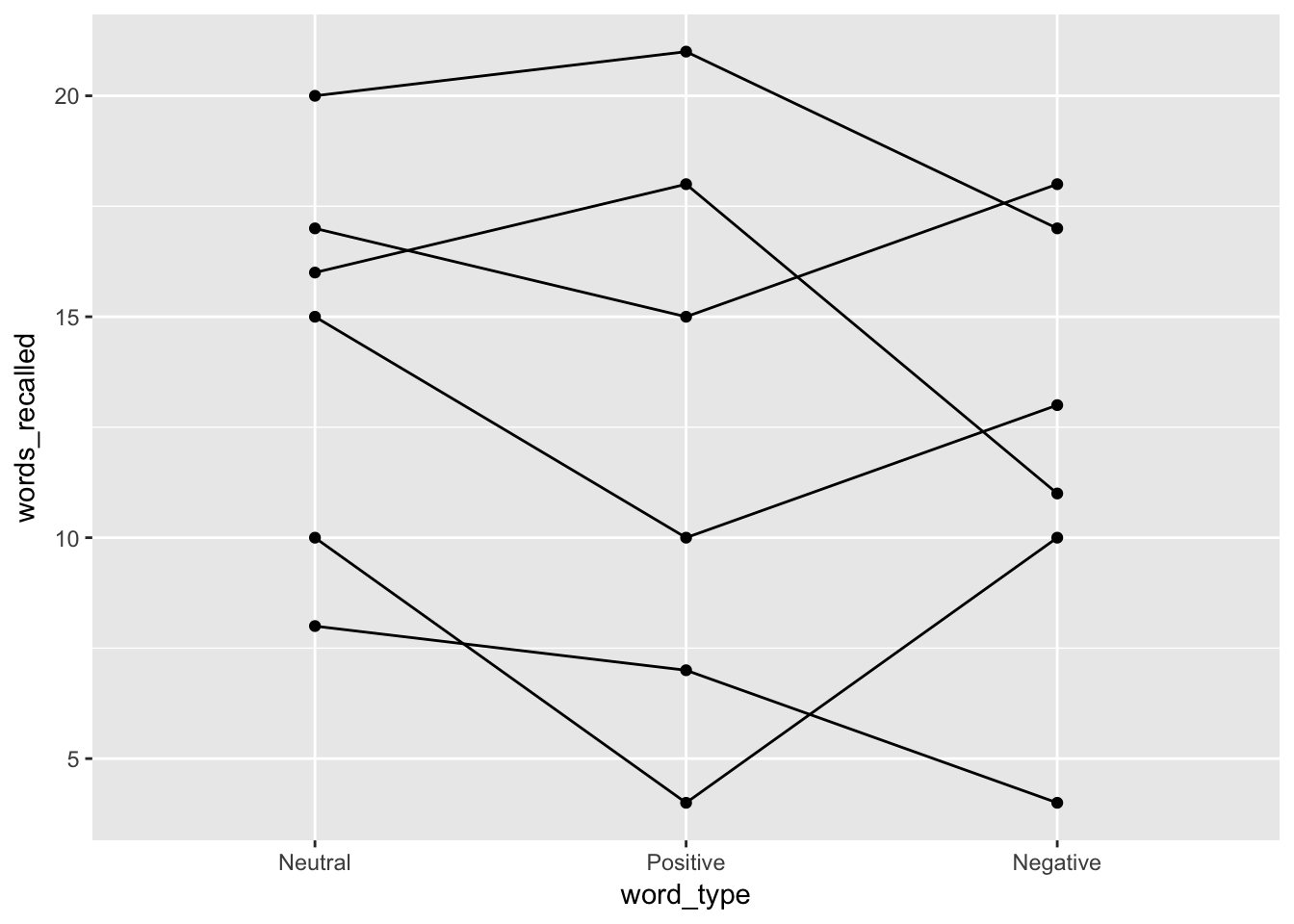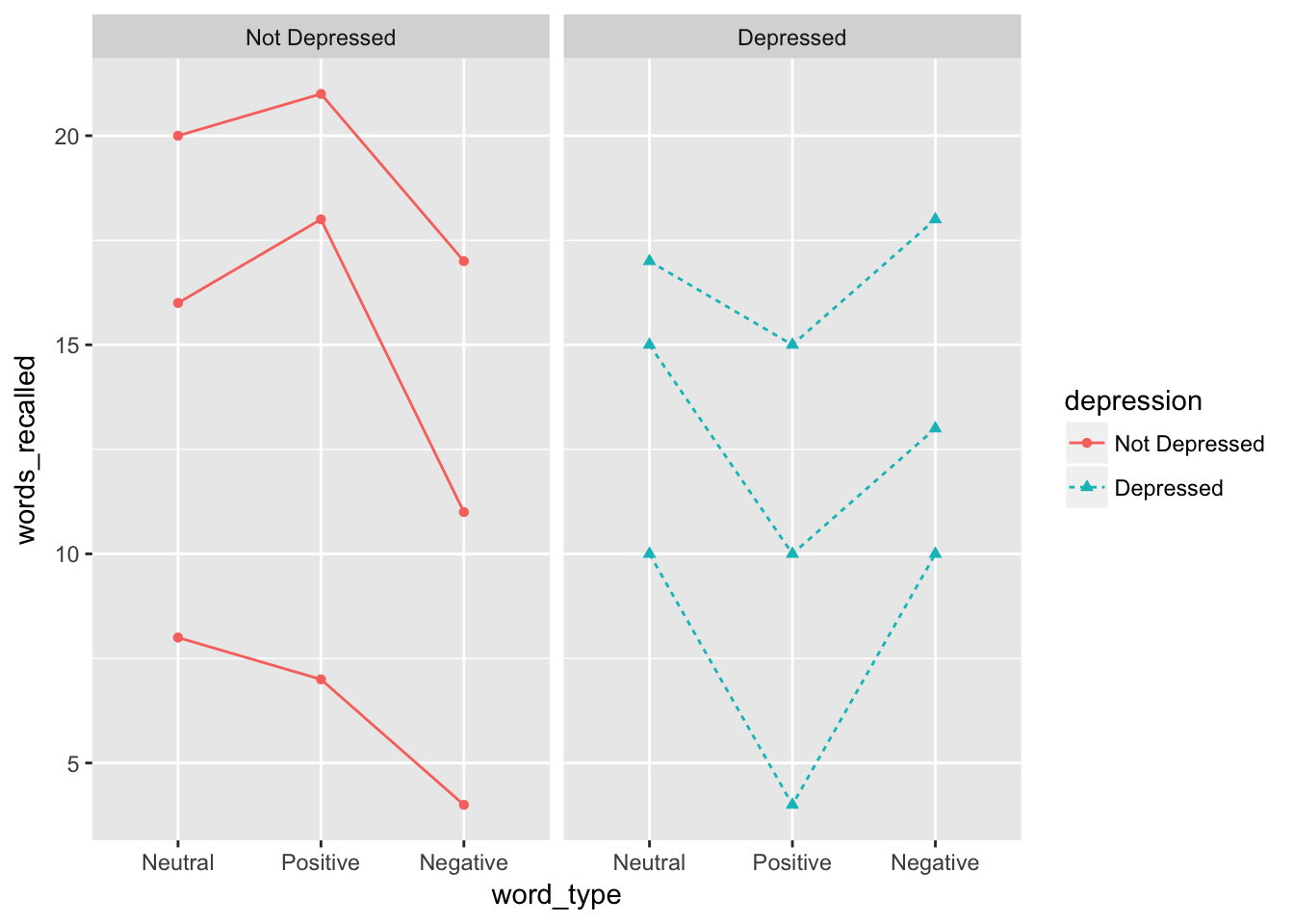## Words Recalled Data Example (Chapter 16a)

### Data Prep

Let’s start with an example of a situation where a mixed ANOVA helps us see patterns that we otherwise miss. To do so, let’s start our `R` code the same way we usually do, with loading our libraries.

``````library(tidyverse)
library(furniture)
library(haven)
library(afex)``````

I input the data as a `tribble` which saves it as a `data.frame` and then cleaned up a few of the important variables.

``````d <- tibble::tribble(
~ID, ~depression, ~word_type, ~words_recalled,
1,           0,          1,              20,
2,           0,          1,              16,
3,           0,          1,               8,
4,           1,          1,              17,
5,           1,          1,              15,
6,           1,          1,              10,
1,           0,          2,              21,
2,           0,          2,              18,
3,           0,          2,               7,
4,           1,          2,              15,
5,           1,          2,              10,
6,           1,          2,               4,
1,           0,          3,              17,
2,           0,          3,              11,
3,           0,          3,               4,
4,           1,          3,              18,
5,           1,          3,              13,
6,           1,          3,              10) %>%
mutate(depression = factor(depression,
labels = c("Not Depressed",
"Depressed"))) %>%
mutate(word_type = factor(word_type,
labels = c("Neutral", "Positive", "Negative")))
d``````

### One-Way RM ANOVA

First, let’s ignore the depression variable and just look at the repeated measures. We do this by using `afex::aov_4()` and then the `summary()` functions as shown below.

``````oneway <- d %>%
afex::aov_4(words_recalled ~ 1 + (word_type|ID),
data = .)
summary(oneway)``````
``````##
## Univariate Type III Repeated-Measures ANOVA Assuming Sphericity
##
##              Sum Sq num Df Error SS den Df F value   Pr(>F)
## (Intercept) 3042.00      1   381.33      5 39.8864 0.001466 **
## word_type     16.33      2    68.33     10  1.1951 0.342453
## ---
## Signif. codes:  0 '***' 0.001 '**' 0.01 '*' 0.05 '.' 0.1 ' ' 1
##
##
## Mauchly Tests for Sphericity
##
##           Test statistic  p-value
## word_type         0.2134 0.045538
##
##
## Greenhouse-Geisser and Huynh-Feldt Corrections
##  for Departure from Sphericity
##
##            GG eps Pr(>F[GG])
## word_type 0.55972     0.3282
##
##              HF eps Pr(>F[HF])
## word_type 0.6077293  0.3309148``````

Here, we see a number of pieces of information, including the sums of squares, F-statistic, and p-value. The p-value suggests that there is not an effect of `word_type` here (p = .342).

Let’s plot this using a spaghetti plot.

``````d %>%
ggplot(aes(word_type, words_recalled, group = ID)) +
geom_line() +
geom_point()``````But we wonder if depression has an effect on the number of words recalled, and it may interact with word_type. Let’s see what that looks like.

``````d %>%
ggplot(aes(word_type, words_recalled, group = ID,
color = depression,
shape = depression,
linetype = depression)) +
geom_line() +
geom_point() +
facet_wrap(~depression)``````Definitely looks like the effect of word_type depends on whether the individual has depression or not. To add a between subjects factor to a repeated measures ANOVA, we are now doing mixed ANOVA (both between and within subjects factors).

### Mixed ANOVA

To run a mixed ANOVA, use `afex::aov_4()` and then the `summary()` functions again but this type with the between subjects factor included.

``````mixed_anova <- d %>%
afex::aov_4(words_recalled ~ depression + (word_type|ID),
data = .)``````
``## Contrasts set to contr.sum for the following variables: depression``
``summary(mixed_anova)``
``````## Warning in summary.Anova.mlm(object\$Anova, multivariate = FALSE): HF eps >
## 1 treated as 1``````
``````##
## Univariate Type III Repeated-Measures ANOVA Assuming Sphericity
##
##                       Sum Sq num Df Error SS den Df F value    Pr(>F)
## (Intercept)          3042.00      1   375.78      4 32.3808 0.0047104 **
## depression              5.56      1   375.78      4  0.0591 0.8198275
## word_type              16.33      2    11.56      8  5.6538 0.0294740 *
## depression:word_type   56.78      2    11.56      8 19.6538 0.0008178 ***
## ---
## Signif. codes:  0 '***' 0.001 '**' 0.01 '*' 0.05 '.' 0.1 ' ' 1
##
##
## Mauchly Tests for Sphericity
##
##                      Test statistic p-value
## word_type                   0.81657 0.73788
## depression:word_type        0.81657 0.73788
##
##
## Greenhouse-Geisser and Huynh-Feldt Corrections
##  for Departure from Sphericity
##
##                      GG eps Pr(>F[GG])
## word_type             0.845    0.03934 *
## depression:word_type  0.845    0.00183 **
## ---
## Signif. codes:  0 '***' 0.001 '**' 0.01 '*' 0.05 '.' 0.1 ' ' 1
##
##                        HF eps   Pr(>F[HF])
## word_type            1.396104 0.0294739587
## depression:word_type 1.396104 0.0008177732``````

The output provides us with a clear significant interaction shown in the first table. Our previous plot helps illustrate what this interaction is telling us about the patterns. However, it is often useful to show what the groups are doing, not the individuals (especially if your sample size is larger than 20). To do that, we can use:

``````mixed_anova %>%
emmeans::emmip(depression ~ word_type)``````